## `1. What products are involved?`

SRV and SRD

`2. What is the purpose of this article?`

To explain how the engineering units behave in the Inksight systems and when the conversion for cup seconds is working.

`3. Which unit is displayed on the InkSight?`

The Inksight software can display 3 different units for viscosity (mPa.s/cP/s) this feature is useful when a user wants to compare their reading with Zahn cups vs Rheonics readings. Measurements can be visualized from the Color Control tab, measurement units are not displayed in the color control tab (just the values).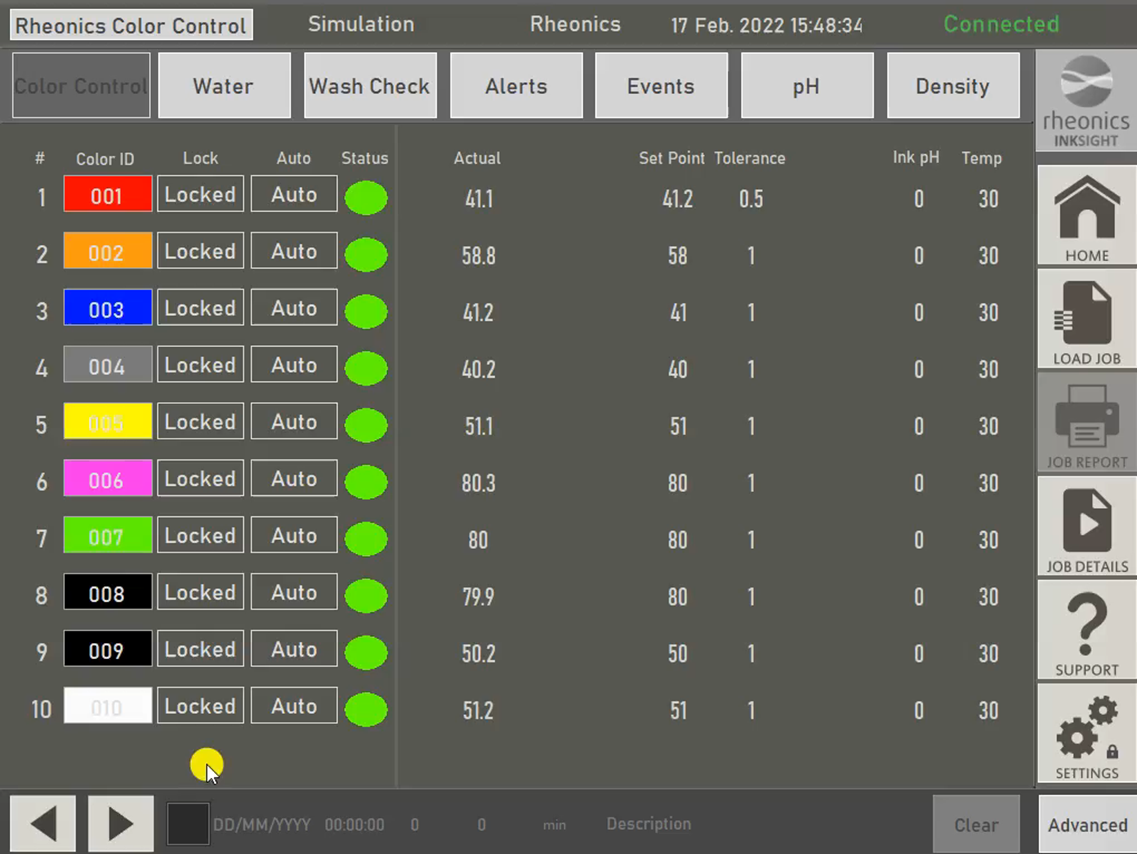Figure 1. Color control tab Inksight software

It is expected for the operators to select one measurement unit and stay with that one when going to the Settings tab. Therefore the expected behavior is as follows:

1. In the settings tabs, check the Units of Viscosity.Figure 2. Viscosity unit selection.

2. If the selected value is cP or mPa.s then the color control tab shows measurements in these units3. In case you switch the viscosity units to Seconds, then the cup-seconds conversion coefficients will be used to calculate the cup-seconds equivalent for the measurement in cP from the sensor.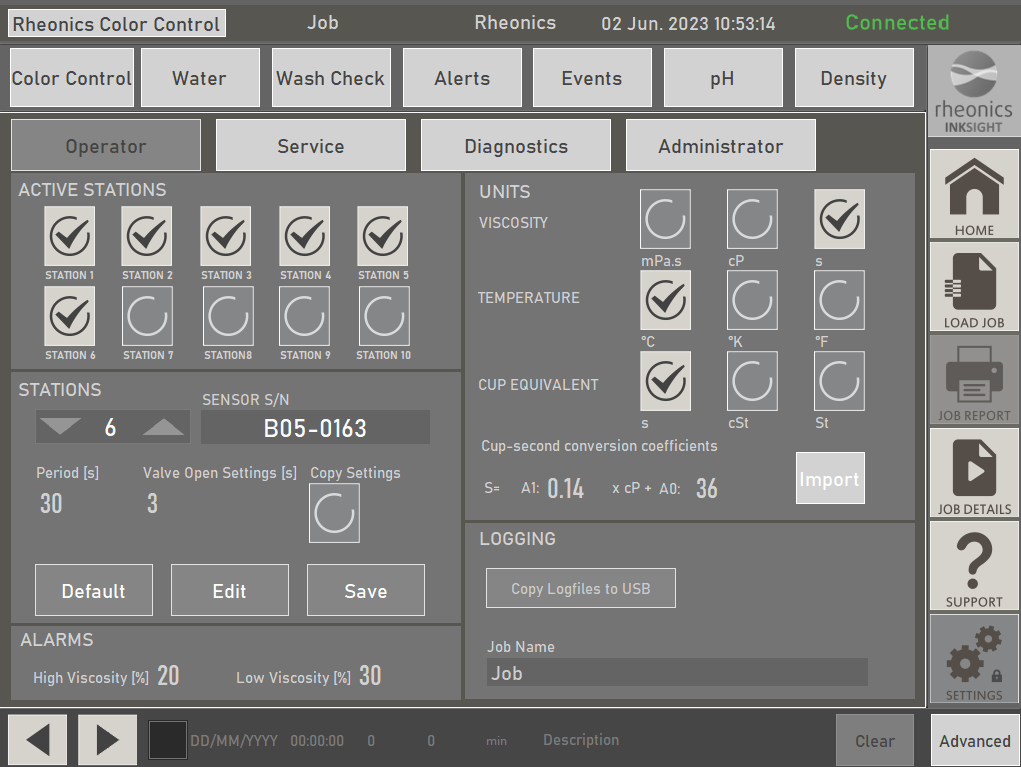Figure 3. Cup-seconds conversion coefficients.

4. The color control tab will display the calculated values in cup seconds.

5. And that's all. By writing these values and having the units selected as cup-seconds, the control values should now show the cup-second equivalent for your cup.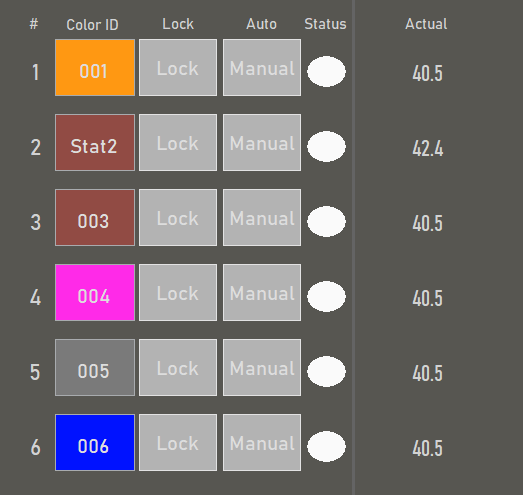Figure 4. Calculated values for cup-second conversion.

6. You can import from a list of pre-calculated coefficients for different units or calculate your own as indicated in this articleConverting inline viscosity measurement to equivalent cup seconds

7. You can input your custom values in the settings. For example, if A1= 0.36 and A0=11, you can write the values directly in the coefficients box: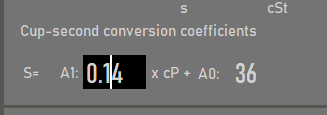Figure 5. A1 coefficients for the cup-second conversion input on the software.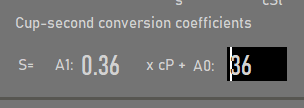Figure 6. A0 coefficients for the cup-second conversion on the software.

8. And that's all. By writing these values and having the units selected as cup-seconds, the control values should now show the cup-second equivalent for your cup.

`Resources`